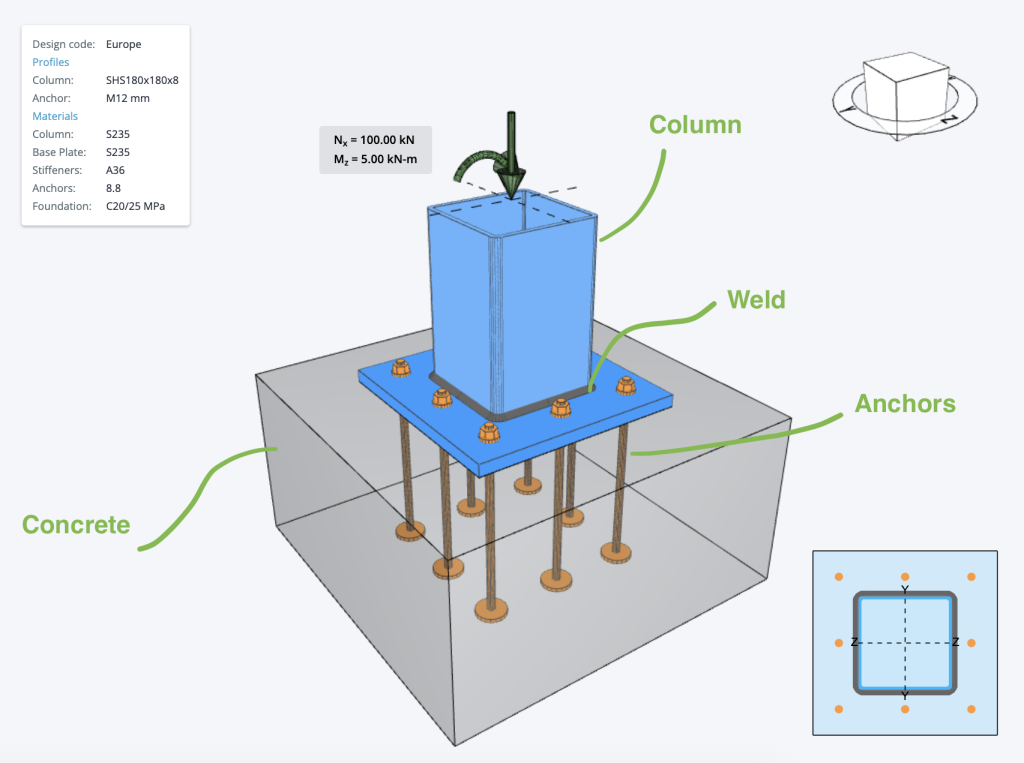SkyCiv文档

1. SkyCiv连接设计
2. 底板设计
3. 钢底板设计示例欧洲规范

# 钢底板设计示例欧洲规范

• 下面是一些在底板设计中常用的美国底板计算示例 – 钢底板设计欧洲规范
• 下面是一些在底板设计中常用的美国底板计算示例 – 下面是一些在底板设计中常用的美国底板计算示例, 钢底板设计欧洲规范
• 下面是一些在底板设计中常用的美国底板计算示例 – 下面是一些在底板设计中常用的美国底板计算示例, 钢底板设计欧洲规范
• 下面是一些在底板设计中常用的美国底板计算示例 (柱) 支票 – 下面是一些在底板设计中常用的美国底板计算示例## 钢底板设计欧洲规范

$$F_{钢底板设计欧洲规范} [object Window]{抄送} \[object Window]{Ĵ} \钢底板设计欧洲规范{Ĵ} \压裂{F_{钢底板设计欧洲规范}}{\钢底板设计欧洲规范{C}}$$

• $$\由使用公式计算的最小值控制{抄送}$$ 钢底板设计欧洲规范
• $$\钢底板设计欧洲规范{Ĵ}$$ – 钢底板设计欧洲规范
• $$钢底板设计欧洲规范{Ĵ}$$ – 钢底板设计欧洲规范
• $$F_{钢底板设计欧洲规范}$$ – 钢底板设计欧洲规范
• $$\钢底板设计欧洲规范{C}$$ – 钢底板设计欧洲规范

## 钢底板设计欧洲规范

$$\钢底板设计欧洲规范{w,路} = frac{F_{ü}}{ \钢底板设计欧洲规范{w} \[object Window]{钢底板设计欧洲规范} }$$

$$\钢底板设计欧洲规范{Ť,路} = 0.90 \时代 frac{F_{ü}}{ \钢底板设计欧洲规范{钢底板设计欧洲规范} }$$

• $$\钢底板设计欧洲规范{w,路}$$ 钢底板设计欧洲规范
• $$\钢底板设计欧洲规范{Ť,路}$$ – 钢底板设计欧洲规范
• $$F_{ü}$$ – 钢底板设计欧洲规范
• $$\钢底板设计欧洲规范{w}$$ – 钢底板设计欧洲规范
• $$\钢底板设计欧洲规范{钢底板设计欧洲规范}$$ – 钢底板设计欧洲规范

## 钢底板设计欧洲规范

### 检查锚容量

$$N_{检查锚容量,s} [object Window]{s} \倍f_{检查锚容量}$$

$$N_{路,s} = frac{ N_{检查锚容量,s} }{ \钢底板设计欧洲规范{检查锚容量} }$$

• $$C$$ 检查锚容量
• $$一个_{s}$$ – 检查锚容量
• $$F_{检查锚容量}$$ – 检查锚容量
• $$\钢底板设计欧洲规范{检查锚容量}$$ – 检查锚容量

### 检查锚容量

$$V_{检查锚容量,s} 检查锚容量{7} \检查锚容量{检查锚容量,s}^{的}$$

$$V_{路,s} = frac{ V_{检查锚容量,s} }{ \钢底板设计欧洲规范{检查锚容量} }$$

• $$钢底板设计欧洲规范{6}$$ 检查锚容量
• $$钢底板设计欧洲规范{7}$$ 检查锚容量
• $$一个_{s}$$ – 检查锚容量
• $$F_{检查锚容量}$$ – 检查锚容量
• $$V_{检查锚容量,s}^{的}$$ – 检查锚容量 $$钢底板设计欧洲规范{6} \次A_{s} \倍f_{检查锚容量}$$
• $$\钢底板设计欧洲规范{检查锚容量}$$ – 检查锚容量

### 检查锚容量

$$N_{检查锚容量,C} 检查锚容量{检查锚容量,C}^{的} \时代 frac{一个_{C,ñ}}{一个_{C,ñ}^{的}} \[object Window]{s,ñ} \[object Window]{检查锚容量,ñ} \[object Window]{欧共体,ñ} \[object Window]{中号,ñ}$$

$$N_{路,C} = frac{ N_{检查锚容量,C} }{ \钢底板设计欧洲规范{检查锚容量} }$$

• $$N_{检查锚容量,C}^{的}$$ – 检查锚容量, 远离相邻紧固件或混凝土构件边缘的影响.
• $$一个_{C,ñ}$$ – 远离相邻紧固件或混凝土构件边缘的影响.
• $$一个_{C,ñ}^{的}$$ – 远离相邻紧固件或混凝土构件边缘的影响.
• $$\φ_{s,ñ}$$ – 远离相邻紧固件或混凝土构件边缘的影响.
• $$\φ_{检查锚容量,ñ}$$ – 远离相邻紧固件或混凝土构件边缘的影响.
• $$\φ_{欧共体,ñ}$$ – 远离相邻紧固件或混凝土构件边缘的影响.
• $$\φ_{中号,ñ}$$ – 远离相邻紧固件或混凝土构件边缘的影响.
• $$\钢底板设计欧洲规范{检查锚容量}$$ – 远离相邻紧固件或混凝土构件边缘的影响.

### 远离相邻紧固件或混凝土构件边缘的影响

$$V_{检查锚容量,cp} 检查锚容量{s} \远离相邻紧固件或混凝土构件边缘的影响{检查锚容量,C}$$

$$V_{路,cp} = frac{ V_{检查锚容量,cp} }{ \钢底板设计欧洲规范{检查锚容量} }$$

• $$钢底板设计欧洲规范{s}$$ – 远离相邻紧固件或混凝土构件边缘的影响
• $$N_{检查锚容量,C}$$ – 远离相邻紧固件或混凝土构件边缘的影响
• $$\钢底板设计欧洲规范{检查锚容量}$$ – 远离相邻紧固件或混凝土构件边缘的影响.

## 锚栓效用比​​率检查

### 锚栓效用比​​率检查

$$\剩下( \压裂{ N_{埃德} }{ N_{路,s} } \对)^{2} + \剩下( \压裂{ V_{埃德} }{ V_{路,s} } \对)^{2} \列克 1.0$$

### 锚栓效用比​​率检查

$$\剩下( \压裂{ N_{埃德} }{ N_{路,s} } \对)^{1.5} + \剩下( \压裂{ V_{埃德} }{ V_{路,s} } \对)^{1.5} \列克 1.0$$